# Leftist Tree / Leftist Heap

A leftist tree or leftist heap is a priority queue implemented with a variant of a binary heap. Every node has an s-value (or rank or distance) which is the distance to the nearest leaf. In contrast to a binary heap (Which is always a complete binary tree), a leftist tree may be very unbalanced.

Below are time complexities of Leftist Tree / Heap.

```  Function       Complexity              Comparison
1) Get Min:       O(1)      [same as both Binary and Binomial]
2) Delete Min:    O(Log n)  [same as both Binary and Binomial]
3) Insert:        O(Log n)  [O(Log n) in Binary and O(1) in
Binomial and O(Log n) for worst case]
4) Merge:         O(Log n)  [O(Log n) in Binomial]```

A leftist tree is a binary tree with properties:

1. Normal Min Heap Property : key(i) >= key(parent(i))
2. Heavier on left side : dist(right(i)) <= dist(left(i)). Here, dist(i) is the number of edges on the shortest path from node i to a leaf node in extended binary tree representation (In this representation, a null child is considered as external or leaf node). The shortest path to a descendant external node is through the right child. Every subtree is also a leftist tree and dist( i ) = 1 + dist( right( i ) ).

Example: The below leftist tree is presented with its distance calculated for each node with the procedure mentioned above. The rightmost node has a rank of 0 as the right subtree of this node is null and its parent has a distance of 1 by dist( i ) = 1 + dist( right( i )). The same is followed for each node and their s-value( or rank) is calculated.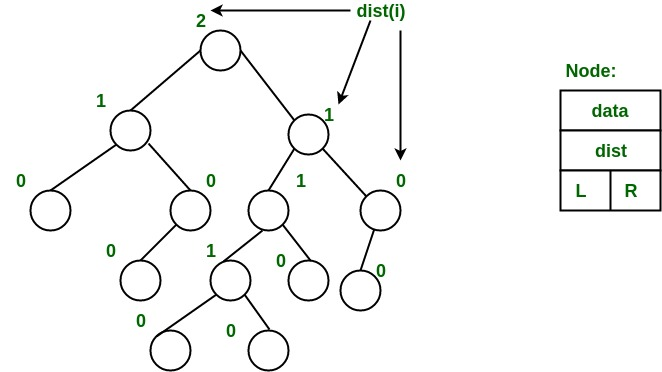From above second property, we can draw two conclusions :

1. The path from root to rightmost leaf is the shortest path from root to a leaf.
2. If the path to rightmost leaf has x nodes, then leftist heap has atleast 2x – 1 nodes. This means the length of path to rightmost leaf is O(log n) for a leftist heap with n nodes.

Operations :

1. The main operation is merge().
2. deleteMin() (or extractMin() can be done by removing root and calling merge() for left and right subtrees.
3. insert() can be done be create a leftist tree with single key (key to be inserted) and calling merge() for given tree and tree with single node.

Idea behind Merging :
Since right subtree is smaller, the idea is to merge right subtree of a tree with other tree. Below are abstract steps.

1. Put the root with smaller value as the new root.
2. Hang its left subtree on the left.
3. Recursively merge its right subtree and the other tree.
4. Before returning from recursion:
– Update dist() of merged root.
– Swap left and right subtrees just below root, if needed, to keep leftist property of merged
result

Detailed Steps for Merge:

1. Compare the roots of two heaps.
2. Push the smaller key into an empty stack, and move to the right child of smaller key.
3. Recursively compare two keys and go on pushing the smaller key onto the stack and move to its right child.
4. Repeat until a null node is reached.
5. Take the last node processed and make it the right child of the node at top of the stack, and convert it to leftist heap if the properties of leftist heap are violated.
6. Recursively go on popping the elements from the stack and making them the right child of new stack top.

Example:
Consider two leftist heaps given below: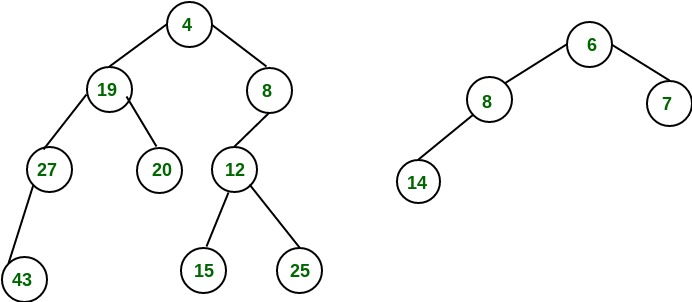Merge them into a single leftist heap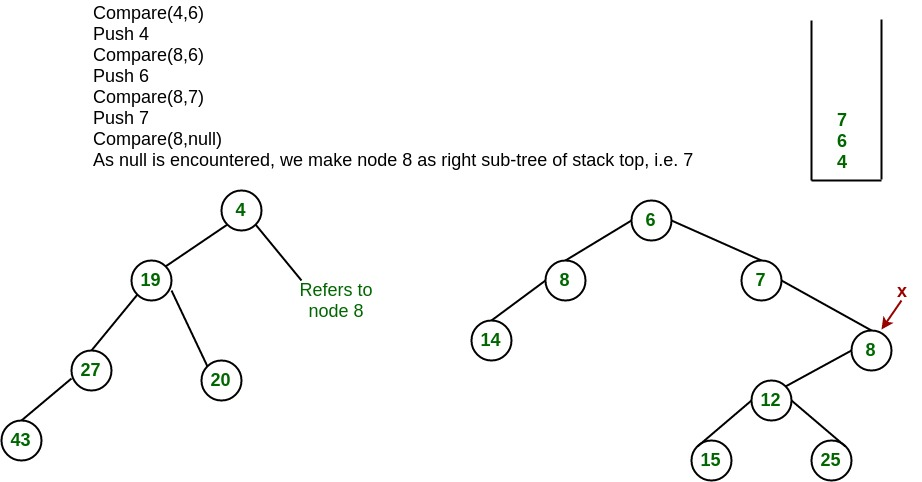The subtree at node 7 violates the property of leftist heap so we swap it with the left child and retain the property of leftist heap.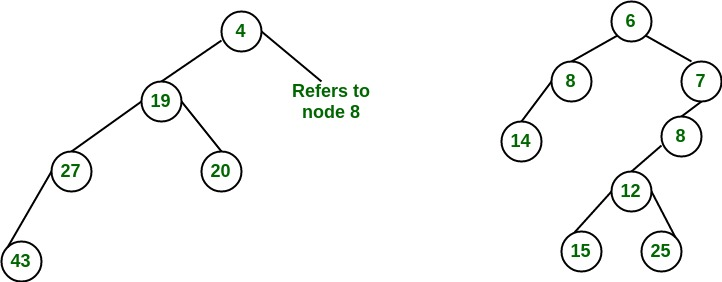Convert to leftist heap. Repeat the process

The worst case time complexity of this algorithm is O(log n) in the worst case, where n is the number of nodes in the leftist heap.

Another example of merging two leftist heap: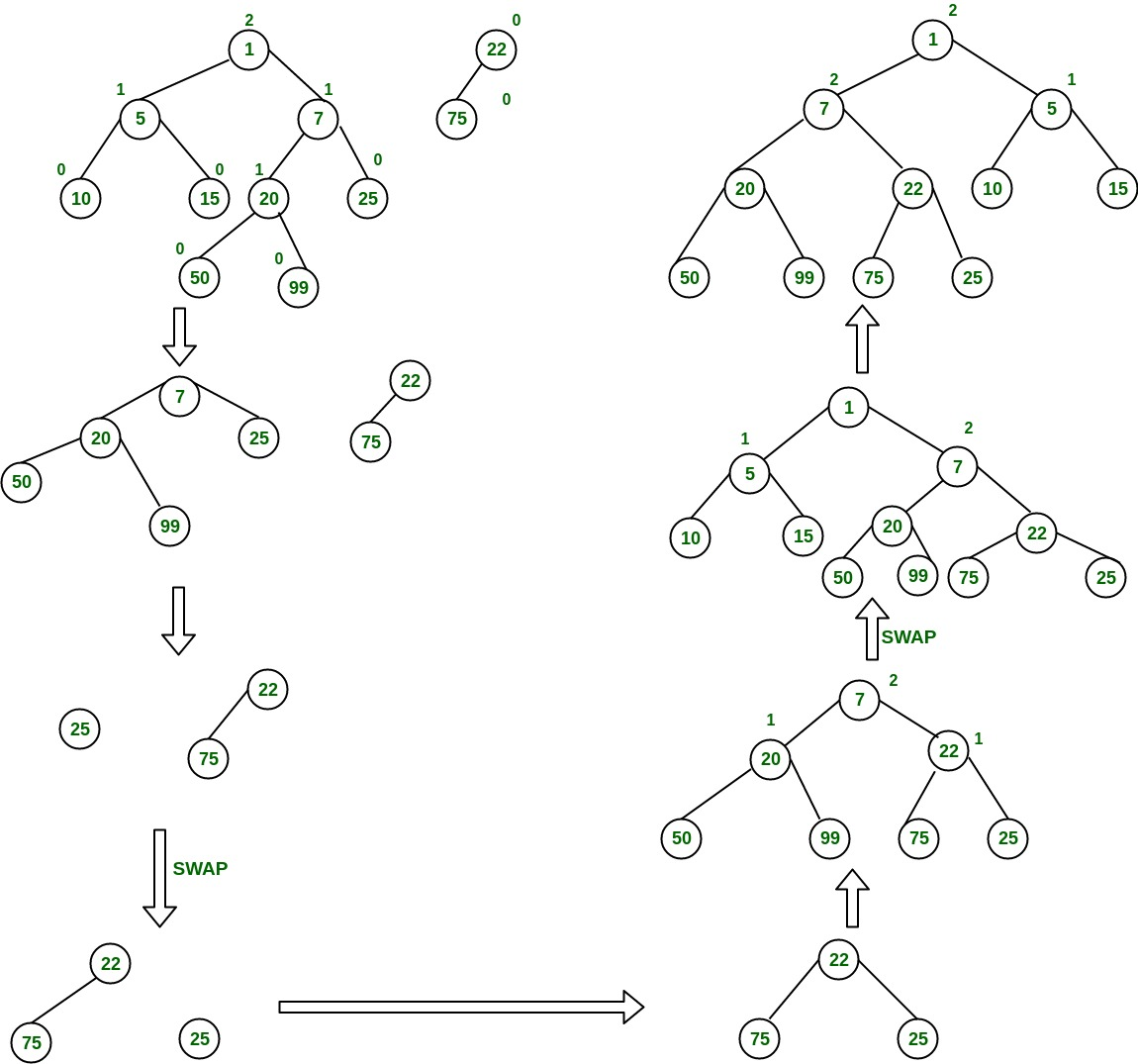Implementation of leftist Tree / leftist Heap:

 `//C++ program for leftist heap / leftist tree ` `#include ` `using` `namespace` `std; ` ` `  `// Node Class Declaration ` `class` `LeftistNode ` `{ ` `public``: ` `    ``int` `element; ` `    ``LeftistNode *left; ` `    ``LeftistNode *right; ` `    ``int` `dist; ` `    ``LeftistNode(``int` `& element, LeftistNode *lt = NULL, ` `                ``LeftistNode *rt = NULL, ``int` `np = 0) ` `    ``{ ` `        ``this``->element = element; ` `        ``right = rt; ` `        ``left = lt, ` `        ``dist = np; ` `    ``} ` `}; ` ` `  `//Class Declaration ` `class` `LeftistHeap ` `{ ` `public``: ` `    ``LeftistHeap(); ` `    ``LeftistHeap(LeftistHeap &rhs); ` `    ``~LeftistHeap(); ` `    ``bool` `isEmpty(); ` `    ``bool` `isFull(); ` `    ``int` `&findMin(); ` `    ``void` `Insert(``int` `&x); ` `    ``void` `deleteMin(); ` `    ``void` `deleteMin(``int` `&minItem); ` `    ``void` `makeEmpty(); ` `    ``void` `Merge(LeftistHeap &rhs); ` `    ``LeftistHeap & operator =(LeftistHeap &rhs); ` `private``: ` `    ``LeftistNode *root; ` `    ``LeftistNode *Merge(LeftistNode *h1, ` `                       ``LeftistNode *h2); ` `    ``LeftistNode *Merge1(LeftistNode *h1, ` `                        ``LeftistNode *h2); ` `    ``void` `swapChildren(LeftistNode * t); ` `    ``void` `reclaimMemory(LeftistNode * t); ` `    ``LeftistNode *clone(LeftistNode *t); ` `}; ` ` `  `// Construct the leftist heap ` `LeftistHeap::LeftistHeap() ` `{ ` `    ``root = NULL; ` `} ` ` `  `// Copy constructor. ` `LeftistHeap::LeftistHeap(LeftistHeap &rhs) ` `{ ` `    ``root = NULL; ` `    ``*``this` `= rhs; ` `} ` ` `  `// Destruct the leftist heap ` `LeftistHeap::~LeftistHeap() ` `{ ` `    ``makeEmpty( ); ` `} ` ` `  `/* Merge rhs into the priority queue. ` `rhs becomes empty. rhs must be different ` `from this.*/` `void` `LeftistHeap::Merge(LeftistHeap &rhs) ` `{ ` `    ``if` `(``this` `== &rhs) ` `        ``return``; ` `    ``root = Merge(root, rhs.root); ` `    ``rhs.root = NULL; ` `} ` ` `  `/* Internal method to merge two roots. ` ` ``Deals with deviant cases and calls recursive Merge1.*/` `LeftistNode *LeftistHeap::Merge(LeftistNode * h1, ` `                                ``LeftistNode * h2) ` `{ ` `    ``if` `(h1 == NULL) ` `        ``return` `h2; ` `    ``if` `(h2 == NULL) ` `        ``return` `h1; ` `    ``if` `(h1->element < h2->element) ` `        ``return` `Merge1(h1, h2); ` `    ``else` `        ``return` `Merge1(h2, h1); ` `} ` ` `  `/* Internal method to merge two roots. ` ` ``Assumes trees are not empty, and h1's root contains ` `  ``smallest item.*/` `LeftistNode *LeftistHeap::Merge1(LeftistNode * h1, ` `                                 ``LeftistNode * h2) ` `{ ` `    ``if` `(h1->left == NULL) ` `        ``h1->left = h2; ` `    ``else` `    ``{ ` `        ``h1->right = Merge(h1->right, h2); ` `        ``if` `(h1->left->dist < h1->right->dist) ` `            ``swapChildren(h1); ` `        ``h1->dist = h1->right->dist + 1; ` `    ``} ` `    ``return` `h1; ` `} ` ` `  `// Swaps t's two children. ` `void` `LeftistHeap::swapChildren(LeftistNode * t) ` `{ ` `    ``LeftistNode *tmp = t->left; ` `    ``t->left = t->right; ` `    ``t->right = tmp; ` `} ` ` `  `/* Insert item x into the priority queue, maintaining ` `  ``heap order.*/` `void` `LeftistHeap::Insert(``int` `&x) ` `{ ` `    ``root = Merge(``new` `LeftistNode(x), root); ` `} ` ` `  `/* Find the smallest item in the priority queue. ` `Return the smallest item, or throw Underflow if empty.*/` `int` `&LeftistHeap::findMin() ` `{ ` `    ``return` `root->element; ` `} ` ` `  `/* Remove the smallest item from the priority queue. ` `Throws Underflow if empty.*/` `void` `LeftistHeap::deleteMin() ` `{ ` `    ``LeftistNode *oldRoot = root; ` `    ``root = Merge(root->left, root->right); ` `    ``delete` `oldRoot; ` `} ` ` `  `/* Remove the smallest item from the priority queue. ` `Pass back the smallest item, or throw Underflow if empty.*/` `void` `LeftistHeap::deleteMin(``int` `&minItem) ` `{ ` `    ``if` `(isEmpty()) ` `    ``{ ` `        ``cout<<``"Heap is Empty"``<left); ` `        ``reclaimMemory(t->right); ` `        ``delete` `t; ` `    ``} ` `} ` ` `  `// Internal method to clone subtree. ` `LeftistNode *LeftistHeap::clone(LeftistNode * t) ` `{ ` `    ``if` `(t == NULL) ` `        ``return` `NULL; ` `    ``else` `        ``return` `new` `LeftistNode(t->element, clone(t->left), ` `                               ``clone(t->right), t->dist); ` `} ` ` `  `//Driver program ` `int` `main() ` `{ ` `    ``LeftistHeap h; ` `    ``LeftistHeap h1; ` `    ``LeftistHeap h2; ` `    ``int` `x; ` `    ``int` `arr[]= {1, 5, 7, 10, 15}; ` `    ``int` `arr1[]= {22, 75}; ` ` `  `    ``h.Insert(arr); ` `    ``h.Insert(arr); ` `    ``h.Insert(arr); ` `    ``h.Insert(arr); ` `    ``h.Insert(arr); ` `    ``h1.Insert(arr1); ` `    ``h1.Insert(arr1); ` ` `  `    ``h.deleteMin(x); ` `    ``cout<< x <

Output:

```1
22
5
```

References:
Wikipedia- Leftist Tree
CSC378: Leftist Trees

This article is contributed by Sheena Kohli and Minal Sunil Parchand. If you like GeeksforGeeks and would like to contribute, you can also write an article using contribute.geeksforgeeks.org or mail your article to contribute@geeksforgeeks.org. See your article appearing on the GeeksforGeeks main page and help other Geeks.

Attention reader! Don’t stop learning now. Get hold of all the important DSA concepts with the DSA Self Paced Course at a student-friendly price and become industry ready.

My Personal Notes arrow_drop_up
Article Tags :
Practice Tags :

2

Please write to us at contribute@geeksforgeeks.org to report any issue with the above content.